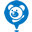# Colopl Inc TSE:3668Colopl Inc
TSE:3668
Price: 590 JPY -1.99%
Updated:

## DCF Value

This DCF valuation model was created byAlpha Spread and was last updated on Oct 1, 2023.

Estimated DCF Value of one3668 stock is 1 046.01 JPY. Compared to the current market price of 590 JPY, the stock is Undervalued by 44%.

DCF Valuation FAQ:
DCF Value
Base Case
1 046.01 JPY
Undervaluation 44%
DCF Value
Price1 046.01
DCF Value
Colopl Inc Competitors:
DCF Valuation
002558
Giant Network Group Co Ltd
603444
G-bits Network Technology Xiamen Co Ltd
800
A8 New Media Group Ltd
063080
Com2uS Holdings Corp
9990
Archosaur Games Inc
047080
Hanbit Soft Inc
AS
Asiasoft Corporation PCL

## Present Value Calculation

The first step in the DCF calculation process is to estimate the company's future free cash flow. Once free cash flow is forecasted, it is discounted at a risk-appropriate discount rate. The resulting value is the present value of the company's free cash flow. You can change any model inputs below.

Available Valuation Models
1 valuation model availableUpdated on Oct 1, 2023
Default Valuation
Other investors haven't shared any valuation models for Colopl Inc.
Model Settings
Operating Model
Discount Rate
4.9%
Forecast Period
5 Years
Terminal Growth
0%
Discount Rate
4.9%
Terminal Growth
0%
Growth Period
5 Years

### DCF Model Base Case ScenarioDCF Model
Currency: JPY
Millions

To view the process of deriving the DCF Value of one share from the estimated Present Value, see the DCF Value Calculation block.

## DCF Value Calculation

Depending on which type of operating model is chosen (equity or whole firm valuation model), the resulting value is either the value of equity or the value of the entire firm. In the case of the latter, to move from the value of the firm to the value of equity, liabilities are subtracted and assets are added.

### Capital Structure From Present Value to DCF ValuePresent Value 66.6B JPY + Cash & Equivalents 57.6B JPY + Investments 9.9B JPY Firm Value 134B JPY - Minority Interest 6m JPY Equity Value 134B JPY / Shares Outstanding 128m 3668 DCF Value 1 046.01 JPY
Undervalued by 44%

To view the process of calculating the Present Value of Colopl Inc' future free cash flow, see the Present Value Calculation block.

## Valuation Analysis

After the valuation process, in order to gain a deeper understanding of the relationship between risk and opportunity, it is useful to study the dynamic of company's valuation under various inputs to the DCF model in use.

Revenue
32.6B 34.4B
Operating Income
5.1B 5.4B
FCFF
2.7B 3.4B

### Sensitivity Analysis DCF Value Sensitivity AnalysisEstimated DCF Value of one3668 stock is 1 046.01 JPY. Compared to the current market price of 590 JPY, the stock is Undervalued by 44%.
1. Present Value Calculation. Using the DCF Operating Model we projectColopl Inc's future free cash flow and discount it at a selected discount rate to calculate its Present Value (66.6B JPY).
2. DCF Value Calculation. We use the company's capital structure to calculate the total Equity Value based on the previously computed Present Value of the free cash flow. Dividing the Equity Value by the number of shares outstanding gives us the DCF Value of 1 046.01 JPY per one3668 share.